Single Phase Half Controlled Converters

# Single Phase Half Controlled Converters - Power Electronics - Electrical Engineering (EE)

Operation and analysis of single phase half controlled converters

Instructional Objectives

On completion the student will be able to

• Draw different topologies of single phase half controlled converter.
• Identify the design implications of each topology.
• Construct the conduction table and thereby draw the waveforms of different system variables in the continuous conduction mode of operation of the converter.
• Analyze the operation of the converter in the continuous conduction mode to find out the average and RMS values of different system variables.
• Find out an analytical condition for continuous conduction relating the load parameters with the firing angle.
• Analyze the operation of the converter in the discontinuous conduction mode of operation.

Introduction

Single phase fully controlled bridge converters are widely used in many industrial applications. They can supply unidirectional current with both positive and negative voltage polarity. Thus they can operate either as a controlled rectifier or an inverter. However, many of the industrial application do not utilize the inverter mode operation capability of the fully controlled converter. In such situations a fully controlled converter with four thyristors and their associated control and gate drive circuit is definitely a more complex and expensive proposition. Single phase fully controlled converters have other disadvantages as well such as relatively poor output voltage (and current for lightly inductive load) form factor and input power factor.
The inverter mode of operation of a single phase fully controlled converter is made possible by the forward voltage blocking capability of the thyristors which allows the output voltage to go negative. The disadvantages of the single phase fully controlled converter are also related to the same capability. In order to improve the output voltage and current form factor the negative excursion of the output voltage may be prevented by connecting a diode across the output as shown in Fig 11.1(a). Here as the output voltage tries to go negative the diode across the load becomes forward bias and clamp the load voltage to zero. Of course this circuit will not be able to operate in the inverter mode. The complexity of the circuit is not reduced, however. For that, two of the thyristors of a single phase fully controlled converter has to be replaced by two diodes as shown in Fig 11.1 (b) and (c) . The resulting converters are called single phase half controlled converters. As in the case of fully controlled converters, the devices T1 and D2 conducts in the positive input voltage half cycle after Tis turned on. As the input voltage passes through negative going zero crossing Dcomes into conduction commutating D2 in Fig 11.1 (b) or T1 in Fig 11.1 (c). The load voltage is thus clamped to zero until T3 is fired in the negative half cycle. As far as the input and output behavior of the circuit is concerned the circuits in Fig 11.1 (b) and (c) are identical although the device designs differs. In Fig 11.1 (c) the diodes carry current for a considerably longer duration than the thyristors. However, in Fig 11.1 (b) both the thyristors and the diodes carry current for half the input cycle. In this lesson the operating principle and characteristics of a single phase half controlled converter will be presented with reference to the circuit in Fig 11.1 (b).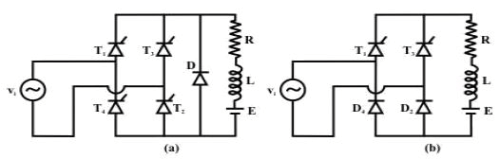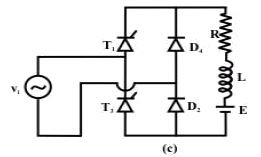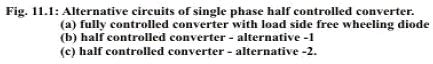Operating principle of a single phase half controlled bridge converter

With reference of Fig 11.1 (b), it can be stated that for any load current to flow one device from the top group (T1 or T3) and one device from the bottom group must conduct. However, T1 T3 or D2 D4 cannot conduct simultaneously. On the other hand T1 D4 and T3 D2 conducts simultaneously whenever T1 or T3 are on and the output voltage tends to go negative. Therefore, there are four operating modes of this converter when current flows through the load. Of course it is always possible that none of the four devices conduct. The load current during such periods will be zero. The operating modes of this converter and the voltage across different devices during these operating modes are shown in the conduction table of Fig 11.2. This table has been prepared with reference to Fig 11.1 (b).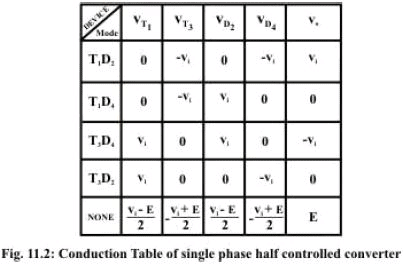It is observed that whenever D2 conducts the voltage across D4 is -vi and whenever D4 conducts the voltage across D2 is vi. Since diodes can block only negative voltage it can be concluded that D2 and Dconducts in the positive and the negative half cycle of the input supply respectively. Similar conclusions can be drawn regarding the conduction of Tand T 3. The operation of the converter can be explained as follows when T1 is fired in the positive half cycle of the input voltage. Load current flows through Tand D2. If at the negative going zero crossing of the input voltage load current is still positive it commutates from D2 to D4 and the load voltage becomes zero. If the load current further continuous till T3 is fired current commutates from T1 to T3. This mode of conduction when the load current always remains above zero is called the continuous conduction mode. Otherwise the mode of conduction becomes discontinuous.

Exercise 11.1

Fill in the blanks(s) with the appropriate word(s)

• In a half controlled converter two ___________________ of a fully controlled converter are replaced by two ___________________.
• Depending on the positions of the ___________________ the half controlled converter can have ___________________ different circuit topologies.
• The input/output waveforms of the two different circuit topologies of a half controlled converter are ___________________ while the device ratings are
•  ___________________.
• A half controlled converter has better output voltage ___________________ compared to a fully controlled converter.
• A half controlled converter has improved input ___________________ compared to a fully controlled converter.

Answer: (i) thyristors, diodes; (ii) diodes, two; (iii) same, different; (iv) form factor; (v) power factor.

2. Find out an expression of the ration of the thyristor to diode RMS current ratings in the single phase half controlled converter topologies of Fig. 11.1(b) & (c). Assume ripple free continuous output current.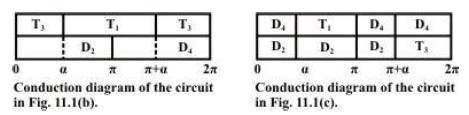In the first conduction diagram the diodes and the thyristors conduct for equal periods, since the load current is constant. The ration of the thyristors to the diode RMS current ratings will be unity for the circuit of Fig 11.1 (b).
From the second conduction diagram the thyristors conduct for π - α radians while the diodes conduct for π + α radians. Since the load current is constant.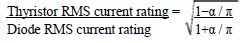in this case

Single phase half controlled converter in the continuous conduction mode

From the conduction table and the discussion in the previous section it can be concluded that the diode D2 and D4 conducts for the positive and negative half cycle of the input voltage waveform respectively. On the other hand T1 starts conduction when it is fired in the positive half cycle of the input voltage waveform and continuous conduction till T3 is fired in the negative half cycle. Fig. 11.3 shows the circuit diagram and the waveforms of a single phase half controlled converter supplying an R – L – E load.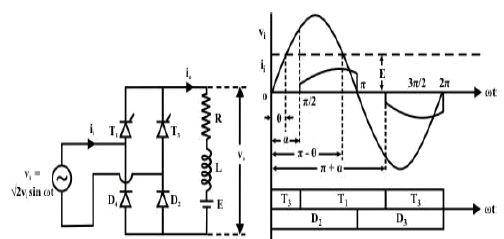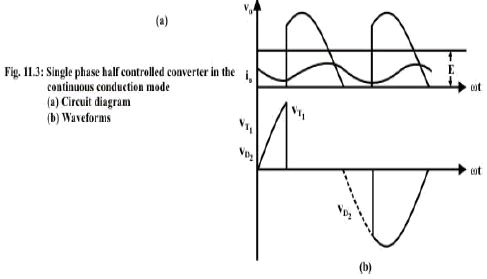Referring to Fig 11.3 (b) T1 D2 starts conduction at ωt = α. Output voltage during this period becomes equal to vi. At ωt = π as vi tends to go negative D4 is forward biased and the load current commutates from D2 to D4 and freewheels through D4 and T1. The output voltage remains clamped to zero till Tis fired at ωt = π + α. The T3 D4 conduction mode continues upto ωt = 2π. Where upon load current again free wheels through T3 and D2 while the load voltage is clamped to zero.

From the discussion in the previous paragraph it can be concluded that the output voltage (hence the output current) is periodic over half the input cycle. Hence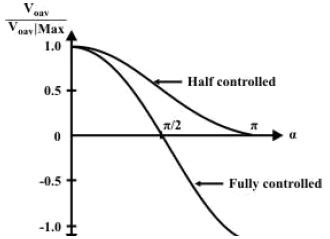Clearly in addition to the average component, the output voltage (and current) contains a large number of harmonic components. The minimum harmonic voltage frequency is twice the input supply frequency. Magnitude of the harmonic voltages can be found by Fourier series analysis of the load voltage and is left as an exercise.

The Fourier series representation of the load current can be obtained from the load voltage by applying superposition principle in the same way as in the case of a fully controlled converter.

However, the closed form expression of io can be found as explained next.

In the period α ≤ ω t ≤ π

The input current ii is given by

ii = i0 for α ≤ ω t ≤ π

ii = - i0 for π + α ≤ ωt ≤ 2π

ii = 0 otherwise                          (11.14)

However, it will be very difficult to find out the characteristic parameters of ii using equation 11.14 since the expression of i0 is considerably complex. Considerable simplification can however be obtained if the actual ii waveform is replaced by a quasisquare wave current waveform with an amplitude of Ioav as shown in Fig 11.5.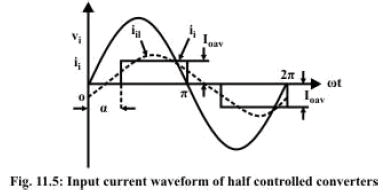Single phase half controlled converter in the discontinuous conduction mode.

So far we have discussed the operating characteristics of a single phase half controlled converter in the continuous conduction mode without identifying the condition required to achieve it. Such a condition exists however and can be found by carefully examining the way this converter works.

Referring to Fig 11.3 (b), when T1 is fired at ωt = α the output voltage (instantaneous value) is larger than the back emf. Therefore, the load current increases till vo becomes equal to E again at ωt = π – θ. There, onwards the load current starts decreasing. Now if io becomes zero before T3 is fired at ωt = π + α the conduction becomes discontinuous. So clearly the condition for continuous conduction will be
If the condition in Eq. 11.22 is violated the conduction will become discontinuous. Clearly, two possibilities exist. In the first case the load current becomes zero before ωt = π. In the second case io continuous beyond ωt = π but becomes zero before ωt = π + α. In both cases however, io starts from zero at ωt = α.

Fig. 11.6 shows the wave forms in these two cases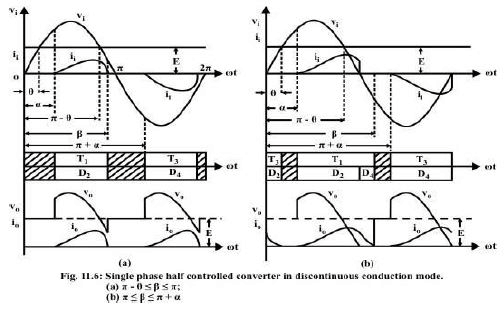Of these two cases the second one will be analyzed in detail here. The analysis of the first case is left as an exercise.
For this case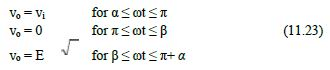However IORMS cannot be computed directly from VORMS. For this the closed form expression for io has to be obtained. This will also help to find out an expression for the conduction angle β.

Fill in the blank(s) with the appropriate word(s).

(iv) At the boundary between continuous and discontinuous conduction the value of the output current at ωt = α is ___________________.
(v) The output voltage and current waveform of a single phase fully controlled and half controlled converter will be same provided the extinction angle β is less than
___________________.
(vi) For the same value of the firing angle the average output voltage of a single phase half controlled converter is ___________________ in the discontinuous conduction mode compared to the continuous conduction mode.
(vii) Single phase half controlled converters are most suitable for loads requiring
___________________ voltage and current.

Answer: (i) zero; (ii) π; (iii) higher; (iv) unidirectional.

2. A single phase half controlled converter charges a 48v 50Ah battery from a 50v, 50Hz single phase supply through a 50mH line inductor. The battery has on interval resistance of 0.1Ω. The
firing angle of the converter is adjusted such that the battery is charged at C/5 rate when it is fully discharged at 42 volts. Find out whether the conduction will be continuous or discontinuous at this condition. Up to what battery voltage will the conduction remain continuous? If the charging current of the battery is to become zero when it is fully charged at 52 volts what should be the value of the firing angle.

Lesson Summary

• Single phase half controlled converters are obtained from fully controlled converters by replacing two thyristors by two diodes.
• Two thyristors of one phase leg or one group (top or bottom) can be replaced resulting in two different topologies of the half controlled converter. From the operational point of view these two topologies are identical.
• In a half controlled converter the output voltage does not become negative and hence the converter cannot operate in the inverter mode.
• For the same firing angle and input voltage the half controlled converter in the continuous conduction mode gives higher output voltage compared to a fully controlled converter.
• For the same input voltage, firing angle and load parameters the half controlled converter has better output voltage and current form factor compared to a fully controlled converter.
• For the same firing angle and load current the half controlled converter in the continuous conduction mode has better input power factor compared to a fully controlled converter.
• Half controlled converters are most favored in applications requiring unidirectional output voltage and current.

Q1. The thyristor T3 of Fig 1.1(b) fails to turn on at the desired instant. Describe how this circuit will work in the presence of the fault.

Q2. A single phase half controlled converter is used to boost the no load speed of a separately excited dc machine by weakening its field supply. At α = 0° the half controlled converter produces the rated field voltage. If the field inductance is large enough to make the field current almost ripple face what will be the input power factor when the dc motor no load speed is bossed to 150%?

Q3. A single phase half controlled converter supplies a 220V, 1500rpm, 20A dc motor from a 230V 50HZ single phase supply. The motor has a armature resistance of 1.0Ω and inductance of 50mH. What will be the operating modes and torques for α = 30°; and speed of 1400 RPM.

The document Single Phase Half Controlled Converters | Power Electronics - Electrical Engineering (EE) is a part of the Electrical Engineering (EE) Course Power Electronics.
All you need of Electrical Engineering (EE) at this link: Electrical Engineering (EE)

## Power Electronics

5 videos|39 docs|66 tests

## FAQs on Single Phase Half Controlled Converters - Power Electronics - Electrical Engineering (EE)

 1. What is a single-phase half controlled converter?Ans. A single-phase half controlled converter is a type of power electronic circuit that converts AC voltage to DC voltage. It uses semiconductor devices such as thyristors to control the flow of current in one direction only. This converter is called "half controlled" because only some of the thyristors are used for control, while the others are used for rectification.
 2. How does a single-phase half controlled converter work?Ans. A single-phase half controlled converter works by using thyristors to control the flow of current in one direction. During the positive half-cycle of the input AC voltage, the thyristors are triggered to conduct and allow current to flow through the load. During the negative half-cycle, the thyristors are non-conducting, and the load is disconnected from the input voltage source. This way, the converter rectifies the AC voltage to DC voltage with controlled output.
 3. What are the advantages of a single-phase half controlled converter?Ans. Some advantages of a single-phase half controlled converter include: - It allows for control of the output voltage by adjusting the firing angle of the thyristors. - It can handle high power levels and is suitable for various applications. - It has a simple circuit configuration, making it cost-effective and reliable. - It provides efficient power conversion with low power losses. - It can be used in both single-phase and three-phase systems, providing versatility.
 4. What are the limitations of a single-phase half controlled converter?Ans. Some limitations of a single-phase half controlled converter are: - It can only control the current flow in one direction, limiting its use in certain applications. - It produces harmonic distortion in the output voltage, which may require additional filtering. - It requires a high voltage rating for the thyristors to handle the peak voltage of the AC input. - The control range of the output voltage is limited by the firing angle range of the thyristors. - It may cause electromagnetic interference (EMI) due to the switching action of the thyristors.
 5. Where are single-phase half controlled converters commonly used?Ans. Single-phase half controlled converters are commonly used in various applications, including: - Variable speed motor drives: They are used to control the speed of single-phase AC motors, such as fans, pumps, and small appliances. - Power supplies: They are used in DC power supplies that require a controlled output voltage, such as battery chargers and welding machines. - Heating systems: They are used in electric heating systems, such as electric furnaces and water heaters, to control the power supplied to the heating elements. - Lighting control: They can be used for dimming or controlling the intensity of single-phase lighting systems, such as incandescent lamps or halogen bulbs.

## Power Electronics

5 videos|39 docs|66 tests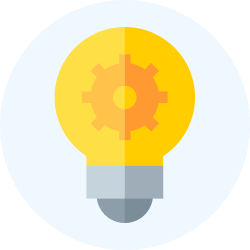Explore Courses for Electrical Engineering (EE) examSignup to see your scores go up within 7 days! Learn & Practice with 1000+ FREE Notes, Videos & Tests.
10M+ students study on EduRev
Track your progress, build streaks, highlight & save important lessons and more!
Related Searches

,

,

,

,

,

,

,

,

,

,

,

,

,

,

,

,

,

,

,

,

,

;